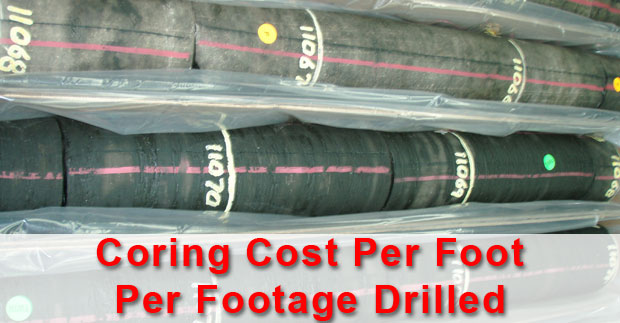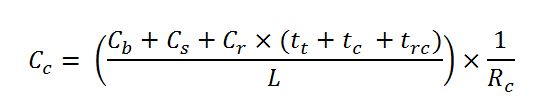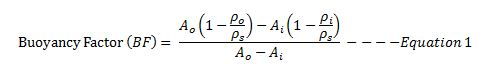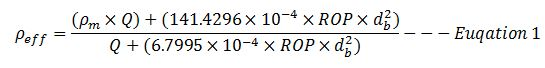## Coring Cost Per Footage Drilled

Coring is a special process to recover wellbore rock in the well.Figure 1 – Core from the well. Credit – Wikipedia

This article will demonstrate how to calculate coring cost per footage recovered.Coring cost per footage recovered is expressed below;## Volume of Cutting Generated While Drilling

While drilling, cuttings are generated every footage drilled and this topic will demonstrate how to determine volume of cutting entering into the wellbore.Figure 1 – Cutting Generated While Drilling

The following formula is used to calculate cutting volume generated while drilling;Where;

Vc is volume of cutting in bbl/hr.

Ø is formation porosity (%).

D is wellbore diameter in inch.

ROP is rate of penetration in feet per hour.

Vc can be presented in several unit as follows;

## Buoyancy Factor with Two Different Fluid Weights in The WellBuoyancy Factor is the factor that is used to compensate loss of weight due to immersion in drilling fluid and you can find more information from this article > buoyancy factor calculation .  In that article, it demonstrates the buoyancy formula only for one fluid in the wellbore. However, this time, we will have the details about buoyancy factor when inside and outside fluid are different.

Buoyancy factor with different fluid inside and outside of tubular is listed below;Where;

Ao is an external area of the component.

Ai is an internal area of the component.

ρo is fluid density in the annulus at the component depth in the wellbore.

ρi is fluid density in the component depth in the wellbore.

ρs is steel weight density. Steel density is 65.4 ppg.

## Maximum ROP Before Fracture Formation

In this topic, we will apply the effective mud density formula to determine maximum ROP before fracturing formation.Figure 1 – Max ROP before Fracturing Formation

These two equations that will be used to determine the maximum ROP are listed below;

Effective mud density due to cuttings in the hole can be determined by the empirical equation below;Where;

ρeff is effective mud density in ppg.

ρm is mud density in ppg.

Q is flow rate in gpm.

ROP is rate of penetration in fph.

db is wellbore diameter or bit diameter in inch.

## Increase In Mud Weight Due To Cutting

Cutting generated while drilling will increase drilling fluid density and it will finally affect equivalent circulating density while drilling. In this topic, we will talk about how to determine mud weight increase due to cutting.Figure 1 – Cutting Increases Mud Density

Effective mud density due to cuttings in the hole can be determined by the empirical equation below;